# SSC Quantitative Aptitude Questions (Day-72)

Dear Aspirants, you can find the Quantitative Aptitude questions with detailed explanations for the SSC exams. Nowadays the competitive level of the exam has been increasing consistently. Due to the great demand for the government job, the level of the toughness reached greater. Candidates have to enhance the preparation process in order to drive in the right path. It doesn’t need to clear the prescribed cutoff. You must have to score good marks more than the cut off marks to get into the final provisional list. Here we have updating the Quantitative Aptitude questions with detailed explanations on a daily basis. You can practice with us and measure your level of preparation. According to that you can sculpt yourself in a proper way. SSC aspirants kindly make use of it and grab your success in your career.

Start Quiz

1) If x = 17 – 418, then find the value of x+1/√x?

(a) 7√2

(b) 9

(c) 22

(d) 6

2) If a2 + b2 + c2 +
1/a2 +1/b2 +1/c2 =6, then what is the value of a2 + b2 + c2?

(a) 3

(b) 6

(c) –3

(d) 2

3) If 3x2 –9x + 3 = 0, then what is the value of
(x+1/x)3?

(a) 9

(b) 729

(c) 81

(d) 27

4) If x
1/x = 3, then what is the value of x3 –1/x3 ?

(a) 36

(b) 21

(c) 9

(d) 27

5) If x2 – 9x – 1 = 0, then what is the value of x2 +
1/x2  + 5x – 5/x?

(a) 115

(b) 128

(c) 124

(d) 133

6) A circle passing through points Q and R of triangle PQR, cut the sides PQ and PR at point X and Y respectively. If PQ = PR, then what is the value (indegrees) of ∠PRQ + ∠QXY?

(a) 120

(b) 150

(c) 240

(d) 180

7) A, B and C are the three points on a circle such that ∠ABC = 35° and ∠BAC = 85°. What is the angle (in degrees) subtended by arc AB at the centre ofthe circle?

(a) 60

(b) 90

(c) 135

(d) 120

8) In ΔPQR, S and T are the mid points of sides PQ and PR respectively. If ∠QPR = 45° and ∠PRQ = 55°, then what is the value (in degrees) of ∠QST?

(a) 80

(b) 85

(c) 90

(d) 100

9) AB is a tangent to a circle with centre Oand point B lying on the circle. If the radius of the circle is 7 cm and the length of AB is 24 cm, then what is the length (in cm) of OA?

(a) 25

(b) 26

(c) 28

(d) 31

10) In the given figure, what is the value of
1 +∠2 + ∠3 + ∠4 + ∠5 + ∠6 + ∠7 + ∠8 + ∠9 + ∠10?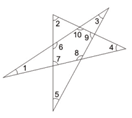(a) 600

(b) 720

(c) 900

(d) 1080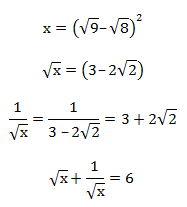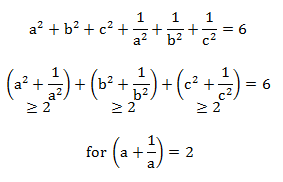a = 1

hence a = b = c = 1

= 1² + 1² + 1²

= 3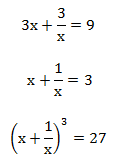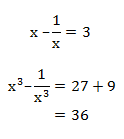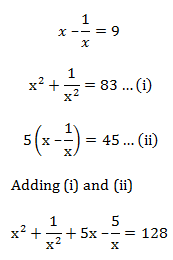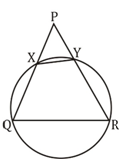∠PRQ & ∠QXY are supplementary angle

∵ QXYR is a cyclic quadrilateral.

∠PRQ + ∠QXY = 180°∠C = 180° – 120° = 60°

∠AOB = 60 × 2 = 120°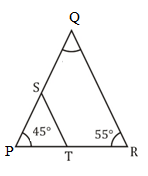From mid-point theorem,

ST ∥ QR

∠SQR = 80°

Hence ∠QST = 100°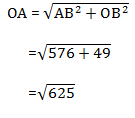= 25 cm

For Points with angle 2, 5 &9it can be assumed that they are making a triangle hence, sum of its angles=  ∠2 + ∠5 + ∠9 = 180°

For Points with angle 6, 10, 4 and 7 it can be assumed that they are making a quadrilateral hence, sum of its angles=   ∠6 + ∠10 + ∠4 + ∠7 = 360°

For Points with angle 1, 3, 8 it can be assumed that they are making another triangle hence, sum of its angles=  ∠1+ ∠3 + ∠8 = 180°

Total angle ∠1 + ∠2 + ∠3 + ∠4 + ∠5 + ∠6 + ∠7 + ∠8 + ∠9 + ∠10 = 720

 Check Here to View SSC CGL / CHSL 2021 Quantitative Aptitude Questions Day – 71 Day – 70 Day – 69